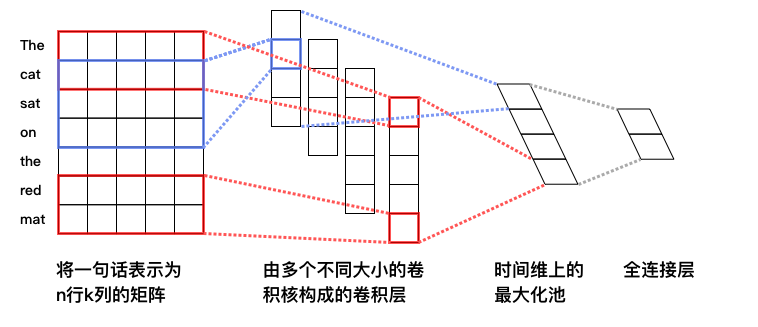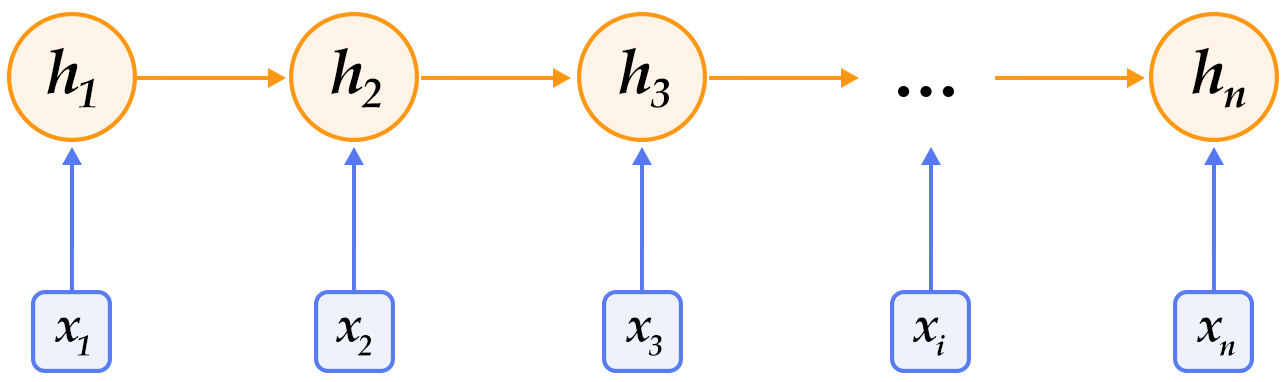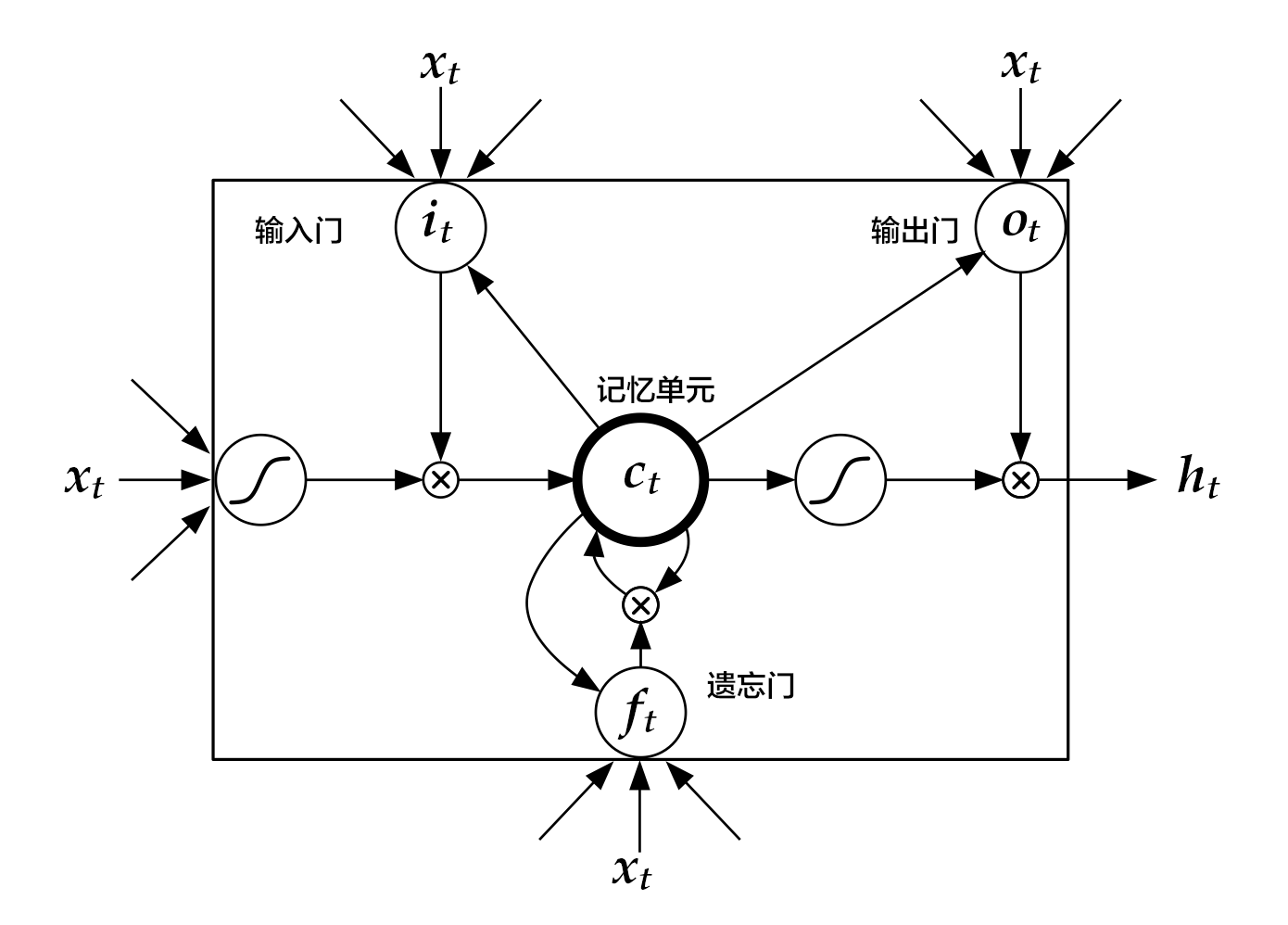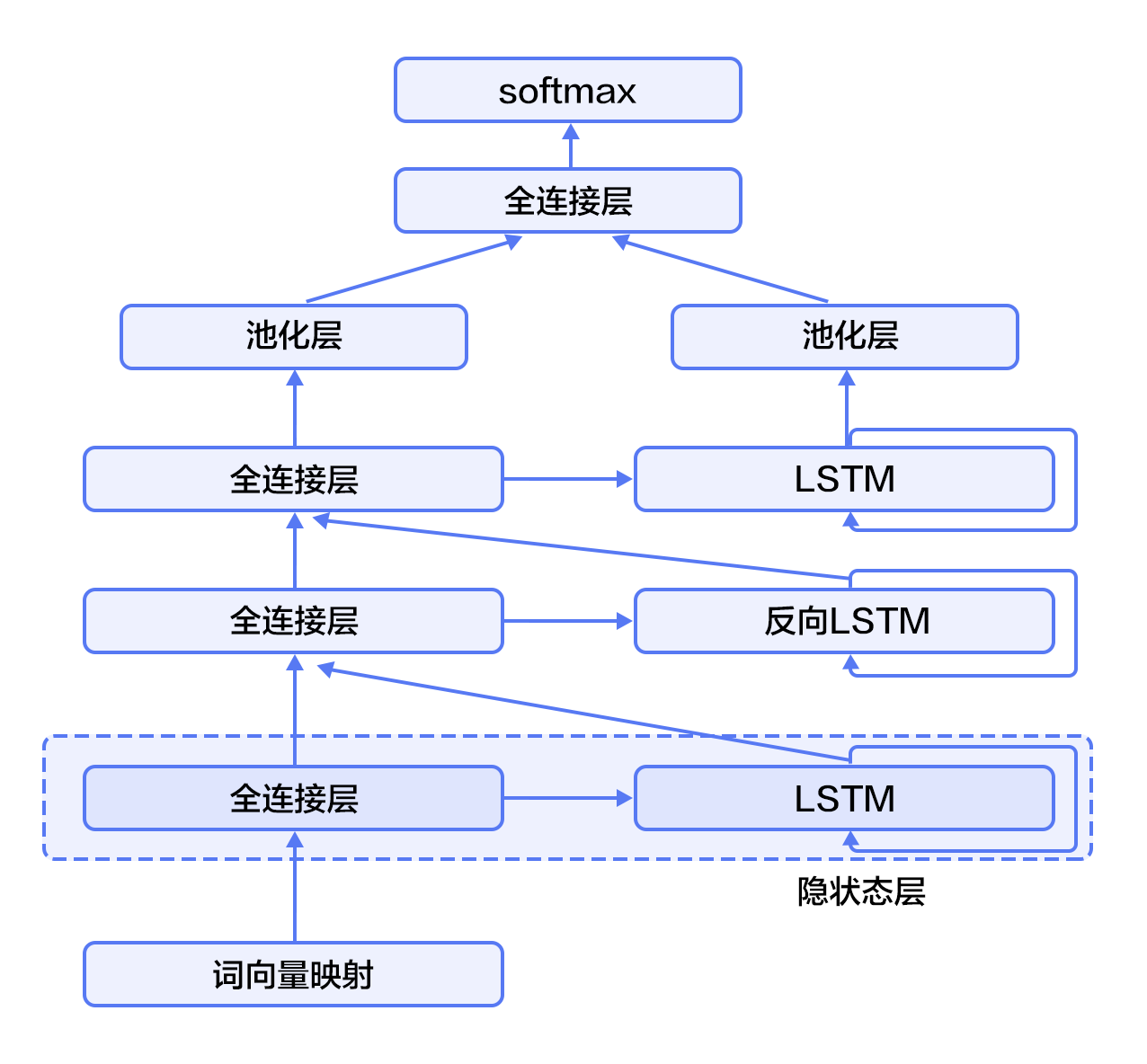# Sentiment Analysis¶

The source code of this tutorial is in book/understand_sentiment. For new users, please refer to Running This Book .

## Background Introduction¶

In natural language processing, sentiment analysis generally refers to judging the emotion expressed by a piece of text. Among them, a piece of text can be a sentence, a paragraph or a document. Emotional state can be two categories, such as (positive, negative), (happy, sad); or three categories, such as (positive, negative, neutral) and so on.The application scenarios of understanding sentiment are very broad, such as dividing the comments posted by users on shopping websites (Amazon, Tmall, Taobao, etc.), travel websites, and movie review websites into positive comments and negative comments; or in order to analyze the user’s overall experience with a product, grab user reviews of the product, and perform sentiment analysis. Table 1 shows an example of understanding sentiment of movie reviews:

In Feng Xiaogang’s movies of the past few years, it is the best one Positive
Very bad feat, like a local TV series Negative
The round-lens lens is full of brilliance, and the tonal background is beautiful, but the plot is procrastinating, the accent is not good, and even though taking an effort but it is hard to focus on the show Negative
The plot could be scored 4 stars. In addition, the angle of the round lens plusing the scenery of Wuyuan is very much like the feeling of Chinese landscape painting. It satisfied me. Positive

Form 1 Sentiment analysis of movie comments

In natural language processing, sentiment is a typical problem of text categorization, which divides the text that needs to be sentiment analysis into its category. Text categorization involves two issues: text representation and classification methods. Before the emergence of the deep learning, the mainstream text representation methods are BOW (bag of words), topic models, etc.; the classification methods are SVM (support vector machine), LR (logistic regression) and so on.

For a piece of text, BOW means that its word order, grammar and syntax are ignored, and this text is only treated as a collection of words, so the BOW method does not adequately represent the semantic information of the text. For example, the sentence “This movie is awful” and “a boring, empty, non-connotative work” have a high semantic similarity in sentiment analysis, but their BOW representation has a similarity of zero. Another example is that the BOW is very similar to the sentence “an empty, work without connotations” and “a work that is not empty and has connotations”, but in fact they mean differently.

The deep learning we are going to introduce in this chapter overcomes the above shortcomings of BOW representation. It maps text to low-dimensional semantic space based on word order, and performs text representation and classification in end-to-end mode. Its performance is significantly improved compared to the traditional method .

## Model Overview¶

The text representation models used in this chapter are Convolutional Neural Networks and Recurrent Neural Networks and their extensions. These models are described below.

### Introduction of Text Convolutional Neural Networks (CNN)¶

We introduced the calculation process of the CNN model applied to text data in the Recommended System section. Here is a simple review.

For a CNN, first convolute input word vector sequence to generate a feature map, and then obtain the features of the whole sentence corresponding to the kernel by using a max pooling over time on the feature map. Finally, the splicing of all the features obtained is the fixed-length vector representation of the text. For the text classification problem, connecting it via softmax to construct a complete model. In actual applications, we use multiple convolution kernels to process sentences, and convolution kernels with the same window size are stacked to form a matrix, which can complete the operation more efficiently. In addition, we can also use the convolution kernel with different window sizes to process the sentence. Figure 3 in the Recommend System section shows four convolution kernels, namely Figure 1 below, with different colors representing convolution kernel operations of different sizes.Figure 1. CNN text classification model

For the general short text classification problem, the simple text convolution network described above can achieve a high accuracy rate . If you want a more abstract and advanced text feature representation, you can construct a deep text convolutional neural network[2, 3].

### Recurrent Neural Network (RNN)¶

RNN is a powerful tool for accurately modeling sequence data. In fact, the theoretical computational power of the RNN is perfected by Turing’ . Natural language is a typical sequence data (word sequence). In recent years, RNN and its derivation (such as long short term memory) have been applied in many natural language fields, such as in language models, syntactic parsing, semantic role labeling (or general sequence labeling), semantic representation, graphic generation, dialogue, machine translation, etc., all perform well and even become the best at present.Figure 2. Schematic diagram of the RNN expanded by time

The RNN expands as time is shown in Figure 2: at the time of $t$, the network reads the $t$th input $x_t$ (vector representation) and the state value of the hidden layer at the previous moment $h_{t- 1}$ (vector representation, $h_0$ is normally initialized to $0$ vector), and calculate the state value $h_t$ of the hidden layer at this moment. Repeat this step until all the inputs have been read. If the function is recorded as $f$, its formula can be expressed as:

$$h_t=f(x_t,h_{t-1})=\sigma(W_{xh}x_t+W_{hh}h_{t-1}+b_h)$$

Where $W_{xh}$ is the matrix parameter of the input to the hidden layer, $W_{hh}$ is the matrix parameter of the hidden layer to the hidden layer, and $b_h$ is the bias vector parameter of the hidden layer, $\sigma$ is the $sigmoid$ function.

When dealing with natural language, the word (one-hot representation) is usually mapped to its word vector representation, and then used as the input $x_t$ for each moment of the recurrent neural network. In addition, other layers may be connected to the hidden layer of the RNN depending on actual needs. For example, you can connect the hidden layer output of a RNN to the input of the next RNN to build a deep or stacked RNN, or extract the hidden layer state at the last moment as a sentence representation and then implement a classification model, etc.

### Long and Short Term Memory Network (LSTM)¶

For longer sequence data, the gradient disappearance or explosion phenomenon is likely to occur during training RNN. To solve this problem, Hochreiter S, Schmidhuber J. (1997) proposed LSTM (long short term memory).

Compared to a simple RNN, LSTM adds memory unit $c$, input gate $i$, forget gate $f$, and output gate $o$. The combination of these gates and memory units greatly enhances the ability of the recurrent neural network to process long sequence data. If the function \is denoted as $F$, the formula is:

$$h_t=F(x_t,h_{t-1})$$

$F$ It is a combination of the following formulas: $$i_t = \sigma{(W_{xi}x_t+W_{hi}h_{t-1}+W_{ci}c_{t-1}+b_i)}$$ $$f_t = \sigma(W_{xf}x_t+W_{hf}h_{t-1}+W_{cf}c_{t-1}+b_f)$$ $$c_t = f_t\odot c_{t-1}+i_t\odot tanh(W_{xc}x_t+W_{hc}h_{t-1}+b_c)$$ $$o_t = \sigma(W_{xo}x_t+W_{ho}h_{t-1}+W_{co}c_{t}+b_o)$$ $$h_t = o_t\odot tanh(c_t)$$ Where $i_t, f_t, c_t, o_t$ respectively represent the vector representation of the input gate, the forget gate, the memory unit and the output gate, the $W$ and $b$ with the angular label are the model parameters, and the $tanh$ is the hyperbolic tangent function. , $\odot$ represents an elementwise multiplication operation. The input gate controls the intensity of the new input into the memory unit $c$, the forget gate controls the intensity of the memory unit to maintain the previous time value, and the output gate controls the intensity of the output memory unit. The three gates are calculated in a similar way, but with completely different parameters.They controll the memory unit $c$ in different ways, as shown in Figure 3:Figure 3. LSTM for time $t$ 

LSTM enhances its ability to handle long-range dependencies by adding memory and control gates to RNN. A similar principle improvement is Gated Recurrent Unit (GRU), which is more concise in design. These improvements are different, but their macro descriptions are the same as simple recurrent neural networks (as shown in Figure 2). That is, the hidden state changes according to the current input and the hidden state of the previous moment, and this process is continuous until the input is processed:

$$h_t=Recurrent(x_t,h_{t-1})$$

Among them, $Recurrent$ can represent a RNN, GRU or LSTM.

### Stacked Bidirectional LSTM¶

For a normal directional RNN, $h_t$ contains the input information before the $t$ time, which is the above context information. Similarly, in order to get the following context information, we can use a RNN in the opposite direction (which will be processed in reverse order). Combined with the method of constructing deep-loop neural networks (deep neural networks often get more abstract and advanced feature representations), we can build a more powerful LSTM-based stack bidirectional recurrent neural network to model time series data.

As shown in Figure 4 (taking three layers as an example), the odd-numbered LSTM is forward and the even-numbered LSTM is inverted. The higher-level LSTM uses the lower LSTM and all previous layers of information as input. The maximum pooling of the highest-level LSTM sequence in the time dimension can be used to obtain a fixed-length vector representation of the text (this representation fully fuses the contextual information and deeply abstracts of the text), and finally we connect the text representation to the softmax to build the classification model.Figure 4. Stacked bidirectional LSTM for text categorization

## Dataset Introduction¶

We use the IMDB sentiment analysis data set as an example. The training and testing IMDB dataset contain 25,000 labeled movie reviews respectively. Among them, the score of the negative comment is less than or equal to 4, and the score of the positive comment is greater than or equal to 7, full score is 10.

aclImdb
|- test
|-- neg
|-- pos
|- train
|-- neg
|-- pos


Paddle implements the automatic download and read the imdb dataset in dataset/imdb.py, and provides API for reading dictionary, training data, testing data, and so on.

## Model Configuration¶

In this example, we implement two text categorization algorithms based on the text convolutional neural network described in the Recommender System section and [Stacked Bidirectional LSTM](#Stacked Bidirectional LSTM). We first import the packages we need to use and define global variables:

from __future__ import print_function
import numpy as np
import sys
import math

CLASS_DIM = 2 #Number of categories for sentiment analysis
EMB_DIM = 128 #Dimensions of the word vector
HID_DIM = 512 #Dimensions of hide layer
STACKED_NUM = 3 #LSTM Layers of the bidirectional stack
BATCH_SIZE = 128 #batch size


### Text Convolutional Neural Network¶

We build the neural network convolution_net, the sample code is as follows. Note that fluid.nets.sequence_conv_pool contains both convolution and pooling layers.

#Textconvolution neural network
def convolution_net(data, input_dim, class_dim, emb_dim, hid_dim):
emb = fluid.embedding(
input=data, size=[input_dim, emb_dim], is_sparse=True)
conv_3 = fluid.nets.sequence_conv_pool(
input=emb,
num_filters=hid_dim,
filter_size=3,
act="tanh",
pool_type="sqrt")
conv_4 = fluid.nets.sequence_conv_pool(
input=emb,
num_filters=hid_dim,
filter_size=4,
act="tanh",
pool_type="sqrt")
prediction = fluid.layers.fc(
input=[conv_3, conv_4], size=class_dim, act="softmax")
return prediction


The network input input_dim indicates the size of the dictionary, and class_dim indicates the number of categories. Here, we implement the convolution and pooling operations using the sequence_conv_pool API.

### Stacked bidirectional LSTM¶

The code of the stack bidirectional LSTM stacked_lstm_net is as follows:

#Stack Bidirectional LSTM
def stacked_lstm_net(data, input_dim, class_dim, emb_dim, hid_dim, stacked_num):

# Calculate word vectorvector
emb = fluid.embedding(
input=data, size=[input_dim, emb_dim], is_sparse=True)

#First stack
#Fully connected layer
fc1 = fluid.layers.fc(input=emb, size=hid_dim)
#lstm layer
lstm1, cell1 = fluid.layers.dynamic_lstm(input=fc1, size=hid_dim)

inputs = [fc1, lstm1]

#All remaining stack structures
for i in range(2, stacked_num + 1):
fc = fluid.layers.fc(input=inputs, size=hid_dim)
lstm, cell = fluid.layers.dynamic_lstm(
input=fc, size=hid_dim, is_reverse=(i % 2) == 0)
inputs = [fc, lstm]

#pooling layer
fc_last = fluid.layers.sequence_pool(input=inputs, pool_type='max')
lstm_last = fluid.layers.sequence_pool(input=inputs, pool_type='max')

#Fully connected layer, softmax prediction
prediction = fluid.layers.fc(
input=[fc_last, lstm_last], size=class_dim, act='softmax')
return prediction


The above stacked bidirectional LSTM abstracts the advanced features and maps them to vectors of the same size as the number of classification. The ‘softmax’ activation function of the last fully connected layer is used to calculate the probability of a certain category.

Again, here we can call any network structure of convolution_net or stacked_lstm_net for training and learning. Let’s take convolution_net as an example.

Next we define the prediction program (inference_program). We use convolution_net to predict the input of fluid.layer.data.

def inference_program(word_dict):
data = fluid.data(
name="words", shape=[None], dtype="int64", lod_level=1)
dict_dim = len(word_dict)
net = convolution_net(data, dict_dim, CLASS_DIM, EMB_DIM, HID_DIM)
# net = stacked_lstm_net(data, dict_dim, CLASS_DIM, EMB_DIM, HID_DIM, STACKED_NUM)
return net


We define training_program here, which uses the result returned from inference_program to calculate the error. We also define the optimization function optimizer_func.

Because it is supervised learning, the training set tags are also defined in fluid.layers.data. During training, cross-entropy is used as a loss function in fluid.layer.cross_entropy.

During the testing, the classifier calculates the probability of each output. The first returned value is specified as cost.

def train_program(prediction):
label = fluid.data(name="label", shape=[None, 1], dtype="int64")
cost = fluid.layers.cross_entropy(input=prediction, label=label)
avg_cost = fluid.layers.mean(cost)
accuracy = fluid.layers.accuracy(input=prediction, label=label)
return [avg_cost, accuracy] #return average cost and accuracy acc

#Optimization function
def optimizer_func():


## Training Model¶

### Defining the training environment¶

Define whether your training is on the CPU or GPU:

use_cuda = False #train on cpu
place = fluid.CUDAPlace(0) if use_cuda else fluid.CPUPlace()


### Defining the data creator¶

The next step is to define a data creator for training and testing. The creator reads in a data of size BATCH_SIZE. Paddle.dataset.imdb.word_dict will provide a size of BATCH_SIZE after each time shuffling, which is the cache size: buf_size.

Note: It may take a few minutes to read the IMDB data, please be patient.

print("Loading IMDB word dict....")

batch_size=BATCH_SIZE)


Word_dict is a dictionary sequence, which is the correspondence between words and labels. You can see it specifically by running the next code:

word_dict


Each line is a correspondence such as (‘limited’: 1726), which indicates that the label corresponding to the word limited is 1726.

### Construction Trainer¶

The trainer requires a training program and a training optimization function.

exe = fluid.Executor(place)
prediction = inference_program(word_dict)
[avg_cost, accuracy] = train_program(prediction)#training program
sgd_optimizer = optimizer_func()# training optimization function
sgd_optimizer.minimize(avg_cost)


This function is used to calculate the result of the model on the test dataset.

def train_test(program, reader):
count = 0
feed_var_list = [
program.global_block().var(var_name) for var_name in feed_order
]
feeder_test = fluid.DataFeeder(feed_list=feed_var_list, place=place)
test_exe = fluid.Executor(place)
accumulated = len([avg_cost, accuracy]) * 
avg_cost_np = test_exe.run(
program=program,
feed=feeder_test.feed(test_data),
fetch_list=[avg_cost, accuracy])
accumulated = [
x + x for x in zip(accumulated, avg_cost_np)
]
count += 1
return [x / count for x in accumulated]


### Providing data and building a main training loop¶

feed_order is used to define the mapping relationship between each generated data and fluid.layers.data. For example, the data in the first column generated by imdb.train corresponds to the words feature.

# Specify the directory path to save the parameters
params_dirname = "understand_sentiment_conv.inference.model"

feed_order = ['words', 'label']
pass_num = 1  #Number rounds of the training loop

# Main loop part of the program
def train_loop(main_program):
# Start the trainer built above
exe.run(fluid.default_startup_program())

feed_var_list_loop = [
main_program.global_block().var(var_name) for var_name in feed_order
]
feeder = fluid.DataFeeder(
feed_list=feed_var_list_loop, place=place)

test_program = fluid.default_main_program().clone(for_test=True)

# Training loop
for epoch_id in range(pass_num):
# Running trainer
metrics = exe.run(main_program,
feed=feeder.feed(data),
fetch_list=[avg_cost, accuracy])

# Testing Results
print('Step {0}, Test Loss {1:0.2}, Acc {2:0.2}'.format(
step_id, avg_cost_test, acc_test))

print("Step {0}, Epoch {1} Metrics {2}".format(
step_id, epoch_id, list(map(np.array,
metrics))))

if step_id == 30:
if params_dirname is not None:
fluid.io.save_inference_model(params_dirname, ["words"],
prediction, exe)# Save model
return


### Training process¶

We print the output of each step in the main loop of the training, and we can observe the training situation.

### Start training¶

Finally, we start the training main loop to start training. The training time is longer. If you want to get the result faster, you can shorten the training time by adjusting the loss value range or the number of training steps at the cost of reducing the accuracy.

train_loop(fluid.default_main_program())


## Application Model¶

### Building a predictor¶

As the training process, we need to create a prediction process and use the trained models and parameters to make predictions. params_dirname is used to store the various parameters in the training process.

place = fluid.CUDAPlace(0) if use_cuda else fluid.CPUPlace()
exe = fluid.Executor(place)
inference_scope = fluid.core.Scope()


### Generating test input data¶

In order to make predictions, we randomly select 3 comments. We correspond each word in the comment to the id in word_dict. If the word is not in the dictionary, set it to unknown. Then we use create_lod_tensor to create the tensor of the detail level. For a detailed explanation of this function, please refer to API documentation.

reviews_str = [
'read the book forget the movie', 'this is a great movie', 'this is very bad'
]
reviews = [c.split() for c in reviews_str]

UNK = word_dict['<unk>']
lod = []
base_shape = []

for c in reviews:
re = np.array([np.int64(word_dict.get(words, UNK)) for words in c])
lod = np.concatenate([lod,re],axis = 0)
base_shape.insert(-1, re.shape)

base_shape = [base_shape]
lod = np.array(lod).astype('int64')
tensor_words = fluid.create_lod_tensor(lod, base_shape, place)


## Applying models and making predictions¶

Now we can make positive or negative predictions for each comment.

with fluid.scope_guard(inference_scope):

[inferencer, feed_target_names,

assert feed_target_names == "words"
results = exe.run(inferencer,
feed={feed_target_names: tensor_words},
fetch_list=fetch_targets,
return_numpy=False)
np_data = np.array(results)
for i, r in enumerate(np_data):
print("Predict probability of ", r, " to be positive and ", r,
" to be negative for review \'", reviews_str[i], "\'")


## Conclusion¶

In this chapter, we take sentiment analysis as an example to introduce end-to-end short text classification using deep learning, and complete all relevant experiments using PaddlePaddle. At the same time, we briefly introduce two text processing models: convolutional neural networks and recurrent neural networks. In the following chapters, we will see the application of these two basic deep learning models on other tasks.